# String x="abc"; 和String x=new String("abc") 区别

String x="abc" 内存会去查找永久代（常量池），如果常量池没有则开辟新的区域，将x指针指向此区域地址，如果有此常量，则直接指向此常量地址。

`````` 	String s3 = new String("hello");
String s4 = "hello";
System.out.println(s3 == s4);// false
System.out.println(s3.equals(s4));// true

String s5 = "hello";
String s6 = "hello";
System.out.println(s5 == s6);// true
System.out.println(s5.equals(s6));// true

String s1 = "hello";
String s2 = "world";
String s=s1+s2;
String s3 = "helloworld";
System.out.println(s3 == s1 + s2); //false 两个变量相加会先在常量池中开辟空间，再相加，再存放到此空间
System.out.println(s == s3);  //false
System.out.println(s3 == "hello" + "world");  //false  //两个常量相加会先相加得到新的常量，再去常量池比较是否存在

# 为什么重写equals时必须重写hashCode方法？

Object的hashcode方法是本地方法，也就是用c语言或c ++实现的，该方法通常将对象的内存地址转换为整数之后返回。 重写的hashCode 实质内容和对象每个属性的hashCode()值相关

`````` public static int hashCode(Object a[]) {
if (a == null)
return 0;

int result = 1;

for (Object element : a)
result = 31 * result + (element == null ? 0 : element.hashCode());

return result;
}

# java 中只有值传递

``````public static void main(String[] args) {
int num1 = 10;
int num2 = 20;

swap(num1, num2);

System.out.println("num1 = " + num1);
System.out.println("num2 = " + num2);
}

public static void swap(int a, int b) {
//调用时候都相当int a=10,b=20
int temp = a;
a = b;
b = temp;

System.out.println("a = " + a);
System.out.println("b = " + b);
}
//输出
a = 20
b = 10
num1 = 10
num2 = 20

`````` public static void main(String[] args) {
int[] arr = { 1, 2, 3, 4, 5 };
System.out.println(arr);
change(arr);
System.out.println(arr);
}

public static void change(int[] array) {
// 将数组的第一个元素变为0
array = 0;
}
//输出
1
0

``````public class Test {

public static void main(String[] args) {
// TODO Auto-generated method stub
Student s1 = new Student("小张");
Student s2 = new Student("小李");
Test.swap(s1, s2);
System.out.println("s1:" + s1.getName());
System.out.println("s2:" + s2.getName());
}

public static void swap(Student x, Student y) {
Student temp = x;
x = y;
y = temp;
System.out.println("x:" + x.getName());
System.out.println("y:" + y.getName());
}
}
//输出
x:小李
y:小张
s1:小张
s2:小李

# hashMap 源码

## 1.7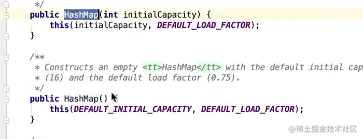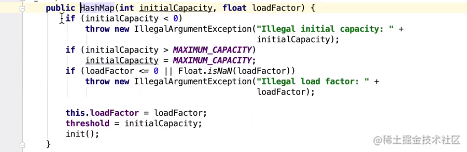1. put过程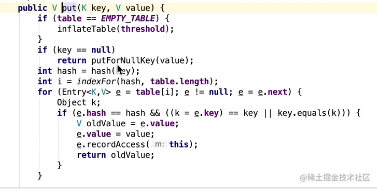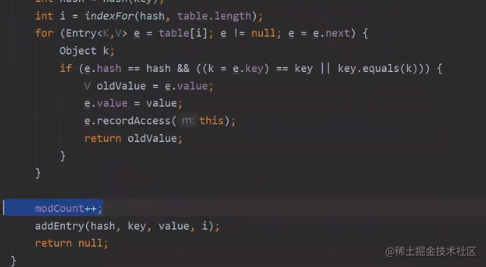1.1 判断数组是否为空，为空 初始化空的数组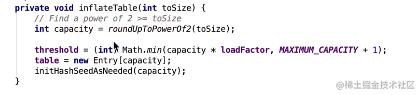new 数组

1.2 hash(key) 方法获得一个hash值 ，内部通过异或和右移让原始hashCode高位参与运算，提高hashCode的散列性

1.3 indexfor 方法得到存放的数组下标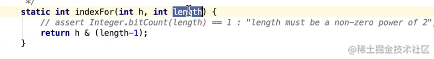1.4 遍历从arr[i]开始的链表 如果当前节点的hash值和刚才计算出的hash值相等且（key的内存地址相等或内容相等），则新的value覆盖旧的value,且返回旧的value

1.5 modCount++ 改变次数+1，和异常有关，在遍历会执行expectCount=modeCount,在hashMap.remove()时modCount++，在判断modeCount==expectCount会抛出异常，是一种fast-fail机制，因为hashMap认为在遍历的过程中增减操作时可能存在风险，不允许这么做。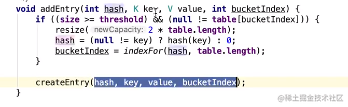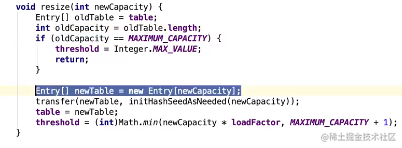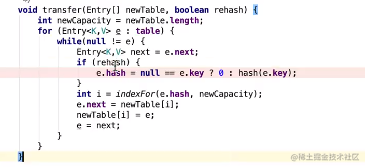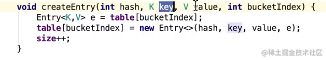# ConcurrentHashMap

## 1.7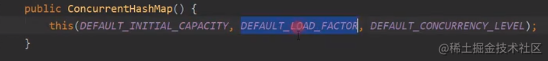DEFAULT_CONCURRENCY_LEVEL ：segment 数量

DEFAULT_INITIAL_CAPACITY ： hashEntry 数量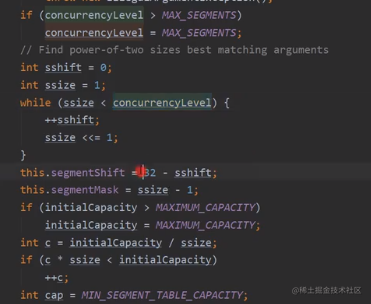segment 数量最多为16个

new Segment[]

put的时候 用cas方式只允许一个线程去修改数组